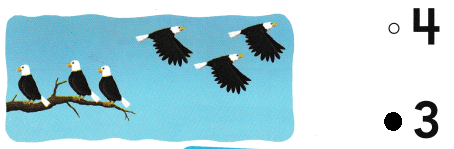# Texas Go Math Kindergarten Lesson 14.4 Answer Key Subtraction Word Problems

Refer to our Texas Go Math Kindergarten Answer Key Pdf to score good marks in the exams. Test yourself by practicing the problems from Texas Go Math Kindergarten Lesson 14.4 Answer Key Subtraction Word Problems.

## Texas Go Math Kindergarten Lesson 14.4 Answer Key Subtraction Word Problems

Essential Question
How can you solve subtraction word problems and complete the subtraction sentence?

Unlock the Problem.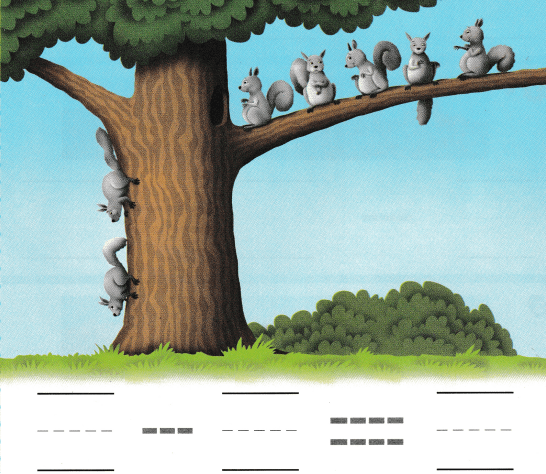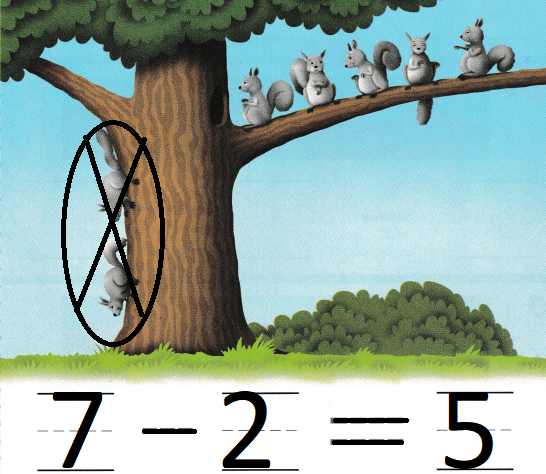Explanation:
There are 7 squirrels on the tree
2 are crawling from the tree
7 – 2 = 5
so, 5 are on the branch.

Directions
Listen to the subtraction word problem about the squirrels. Circle and mark on x on the squirrels that are leaving. How many squirrels are left in the tree? Write and trace the subtraction sentence.

Try Another Problem

Question 1.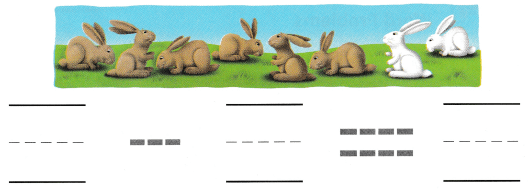Explanation:
There are 8 bunnies
2 bunnies are white
8 – 2 = 6
so, 6 bunnies are yellow.

Question 2.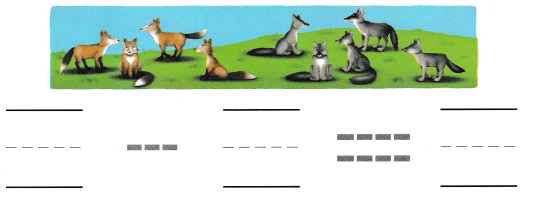Explanation:
There are 9 wolfs
out of 5 are ash
9 – 5 = 4
so, 4 are orange.

Question 3.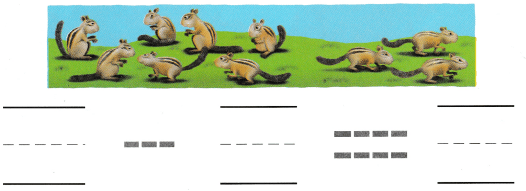Explanation:
There are 10 squirrels
3 run away
10 – 3 = 7
so, 7 squirrels are left.

Directions
1-2. Listen to the subtraction word problem. Write and trace to complete the subtraction sentence. 3. Listen to the subtraction word problem. Circle and mark an X on the chipmunks that run away. Write and trace to complete the subtraction sentence.

Share and Show

Question 4.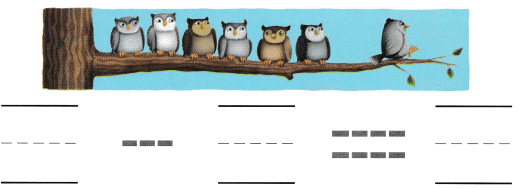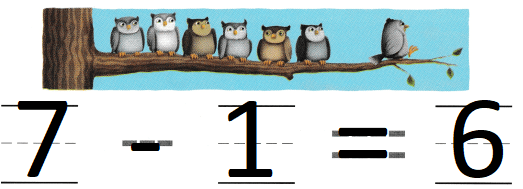Explanation:
There are 7 owls on a tree
1 fly away
7 – 1 = 6
so, owl are left on the tree.

Question 5.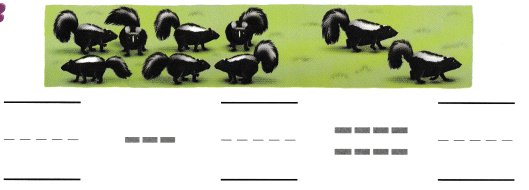Explanation:
There are 9 animals
2 animals run away
9 – 2 = 7
so, animals are left.

Question 6.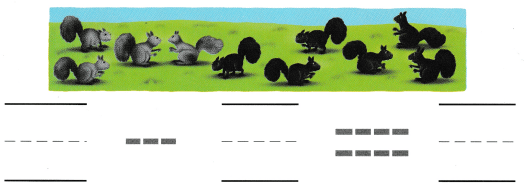Explanation:
There are 10 squirrels
out of 6 are black
10 – 6 = 4
so, 4 are ash.

Question 7.Explanation:
There are 8 birds. Five of the birds are yellow.
The rest of the birds are red.
8 – 5 = 3
3 birds are yellow.

Question 8.Explanation:
There are 7 raccoons.
Three of the raccoons walk away.
7 – 3 = 4
4  raccoons are left

Question 9.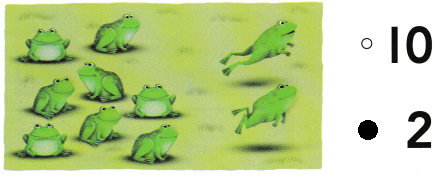Explanation:
There are 10 frogs.
2 frogs hop away
Now there are 8 frogs left,
10 – 2 = 8
8 frogs hopped away

Directions
Choose the correct answer. 7. There are 8 birds. Five of the birds are yellow. The rest of the birds are red. Which number sentence shows how to find the number of red birds? 8. There are 7 raccoons. Three of the raccoons walk away. How many raccoons are left? 9. There ore lo frogs. Some of the frogs hop away. Now there are 8 frogs left, How many frogs hopped away?

### Texas Go Math Kindergarten Lesson 14.4 Homework and Practice

Question 1.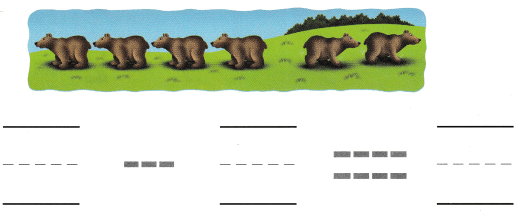Explanation:
There are six bears.
Two bears run away.
6 – 2 = 4
so, 4 bears are there.

Question 2.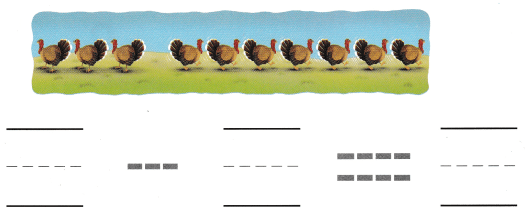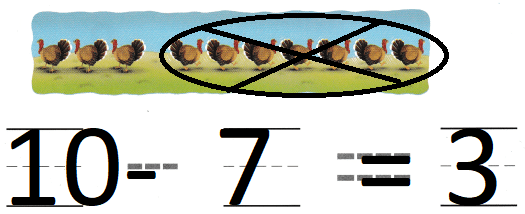Explanation:
There are ten turkeys.
Seven turkeys run away.
10 – 7 = 3
so, 3 turkeys are left.

Directions
Write and trace to complete the subtraction sentence. 1. There are six bears. Two bears run away. Circle and mark on X on the bears that leave. 2. There are ten turkeys. Seven turkeys run away. Circle and mark an X on the turkeys that leave.

Texas Test Prep

Lesson Check

Question 3.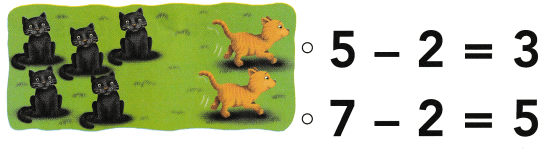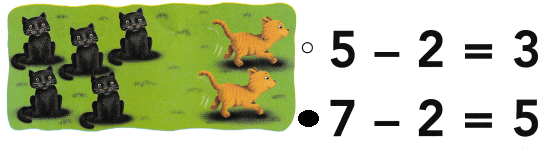Explanation:
There are 7 cats.
Two of them run away
7 – 2 = 5
so, 5 cats are there

Question 4.Explanation:
There are 9 beavers.
Four of them swim away,
9 – 4 = 5
so, 5 beavers on the shore.

Question 5.